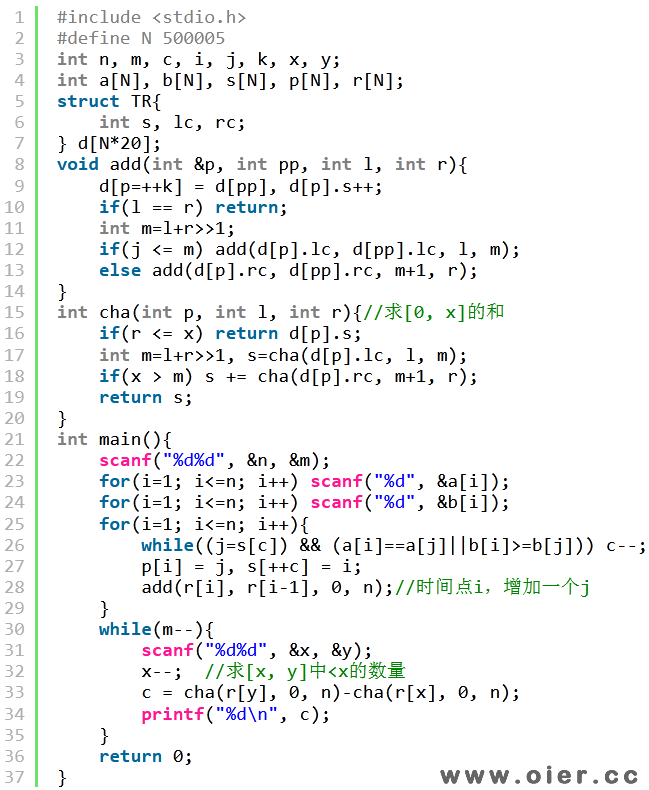361+

## 输入输出格式

### 输出格式

$q$ 行，每行一个自然数表示一组询问的答案。

## 输入输出样例

### 输入样例 #1

10 4
3 1 3 1 2 3 3 2 1 1
10 10 2 9 7 5 4 7 6 1
1 4
7 8
7 10
1 8

### 输出样例 #1

3
2
2
3

## 说明

**【样例解释】**

$(1, 9)$ 的 $a$ 值相同，继续弹栈。此时栈空，$4$ 号二元组入栈，栈为 $\{(1, 9)\}$，该二元组是“成功的”。共有 $3$ 个二元组是“成功的”，因而答案为 $3$。

**【样例 2,3,4】**

**【数据范围与提示】**

| 测试点编号 | 特殊性质 |
| ———— | ————— |
| $1 \sim 3$ | $n,q \leq 1000$ |
| $4 \sim 6$ | $n \leq 5000$ |
| $7 \sim 10$ | $n,q \leq 10^5$ |
| $11 \sim 12$ | $b_i=n-i+1$ |
| $13 \sim 15$ | $a_i=i$ |
| $16 \sim 20$ | 无 |

## 程序实现